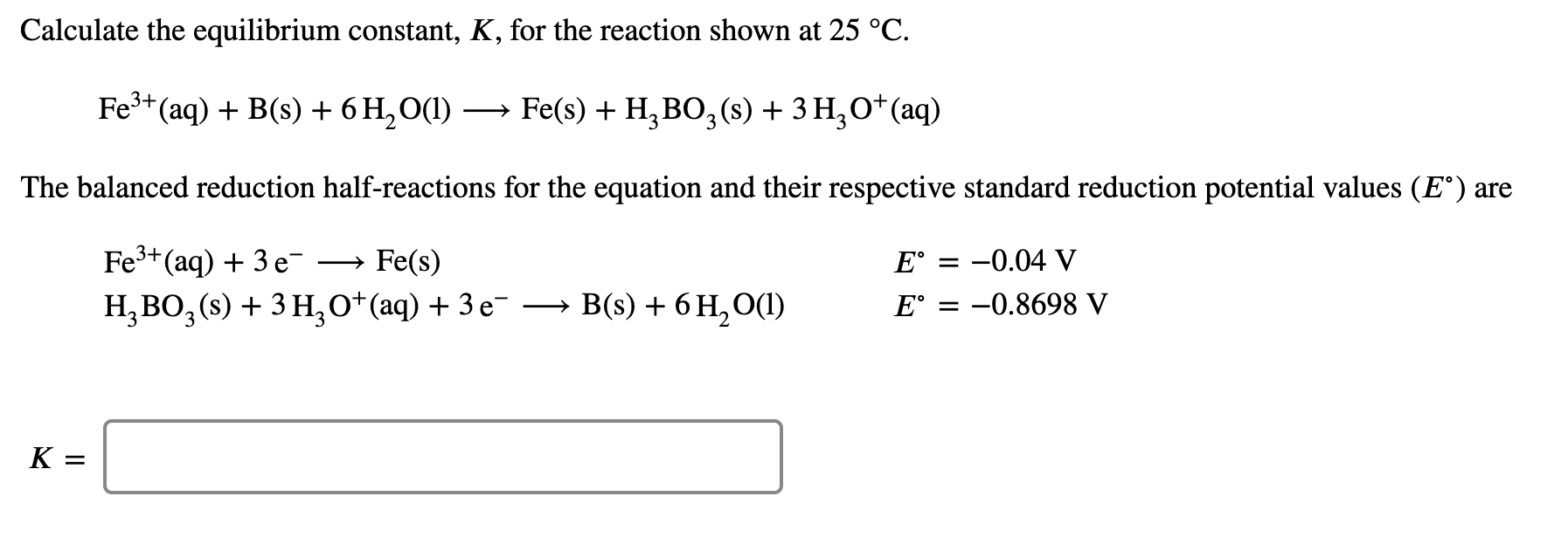# Calculate the equilibrium constant, K for the reaction shown at 25 deg C. Fe 3+ (aq) + B(s) + 6H2O (l)  Fe (s) + H3BO3 (s) + 3H3O+ The balanced reduction half reactions for the equation and their standard reduction potential values (E°) are: Fe3+ (a) + 3e- --> Fe(s) E° = -0.4 V H3BO3 (s) + 3H3O+ + 3e- --> B(s) + 6H2O (l) E° = -0.8698